# Graphing Rational Functions SHRATEY Graphing Rational Functions Using

• Slides: 12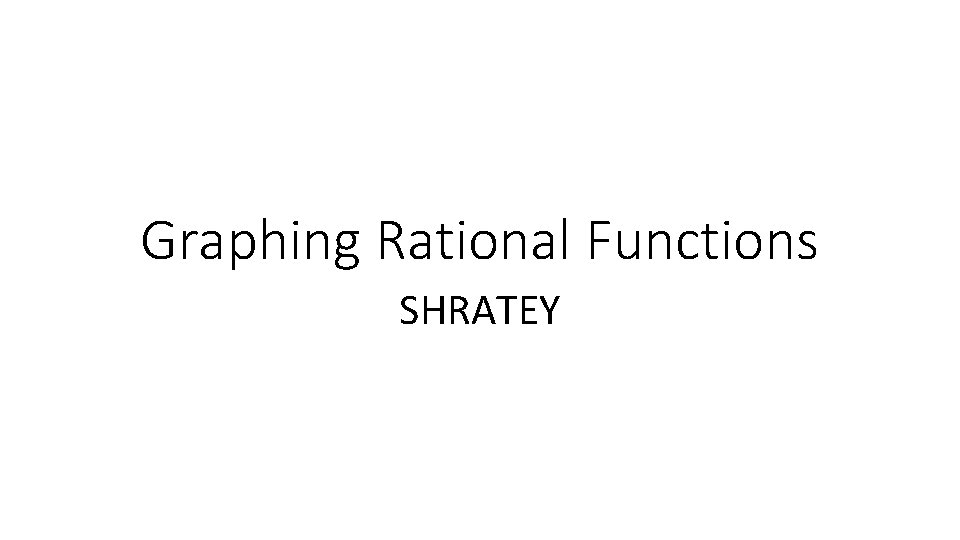Graphing Rational Functions SHRATEY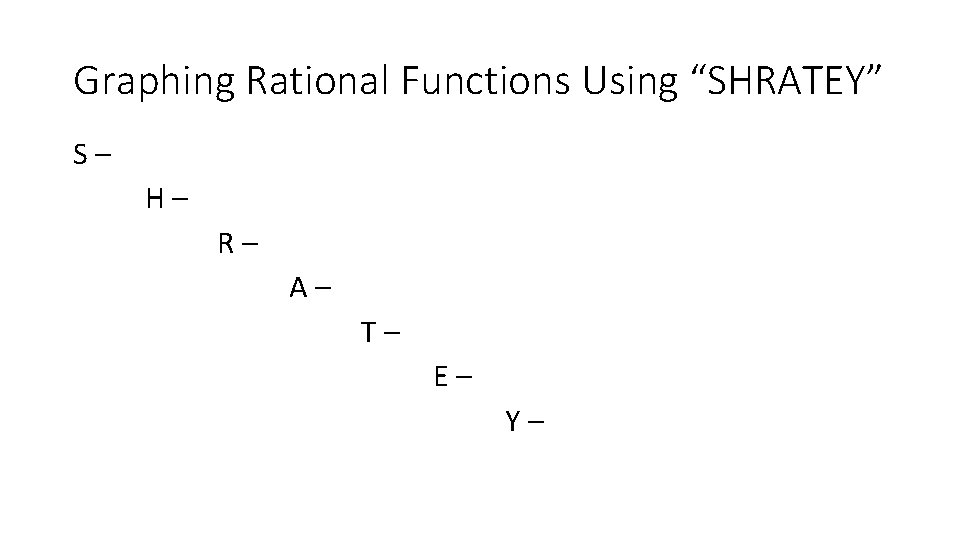Graphing Rational Functions Using “SHRATEY” S– H– R– A– T– E– Y–Graphing Rational Functions Using “SHRATEY” S – Simplify (factor and cancel any common factors) H – identify any holes R – find roots A – find asymptotes T – is the graph tangent or together E – determine end behavior Y – find y-interceptsRATEY Examples Day 3 - #3RATEY Examples Day 3 - #3 Already in simplest terms No factors cancel, this means no holes 3 x+1=0 so x=-1/3 and x+2 = 0 so x = -2 Roots at (-1/3, 0) and (-2, 0) X+1=0 x = -1 There is a vertical asymptote at x = -1 Neither (no squared factors) x +1 Top heavy means oblique asymptote. Multiply out the top to know what we are dividing. The end behavior follows the line y = 3 x+4 Y=((3(0)+1)(0+2))/(0+1) = 2/1= 2 y-intercept is (0, 2) 3 x +4 3 x 2 +7 x +1 -3 x 2 -3 x ↓ 0 4 x +1 -4 x -4 0 -3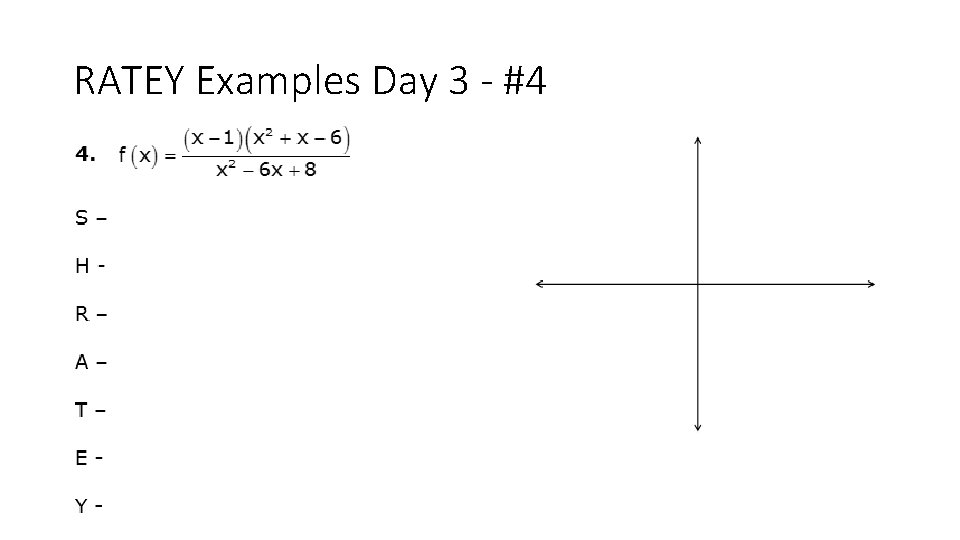RATEY Examples Day 3 - #4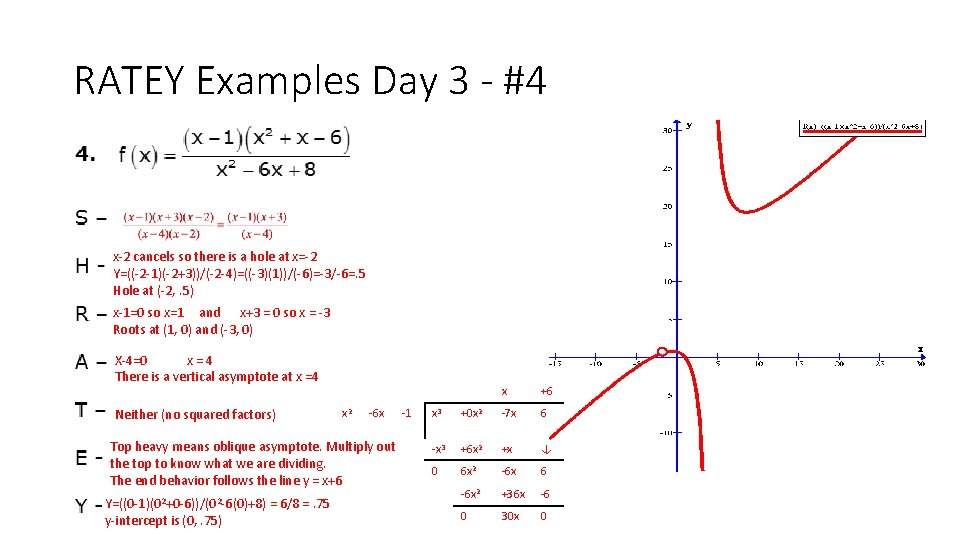RATEY Examples Day 3 - #4 x-2 cancels so there is a hole at x=-2 Y=((-2 -1)(-2+3))/(-2 -4)=((-3)(1))/(-6)=-3/-6=. 5 Hole at (-2, . 5) x-1=0 so x=1 and x+3 = 0 so x = -3 Roots at (1, 0) and (-3, 0) X-4=0 x=4 There is a vertical asymptote at x =4 Neither (no squared factors) x 2 -6 x Top heavy means oblique asymptote. Multiply out the top to know what we are dividing. The end behavior follows the line y = x+6 Y=((0 -1)(02+0 -6))/(02 -6(0)+8) = 6/8 =. 75 y-intercept is (0, . 75) -1 x +6 x 3 +0 x 2 -7 x 6 -x 3 +6 x 2 +x ↓ 0 6 x 2 -6 x 6 -6 x 2 +36 x -6 0 30 x 0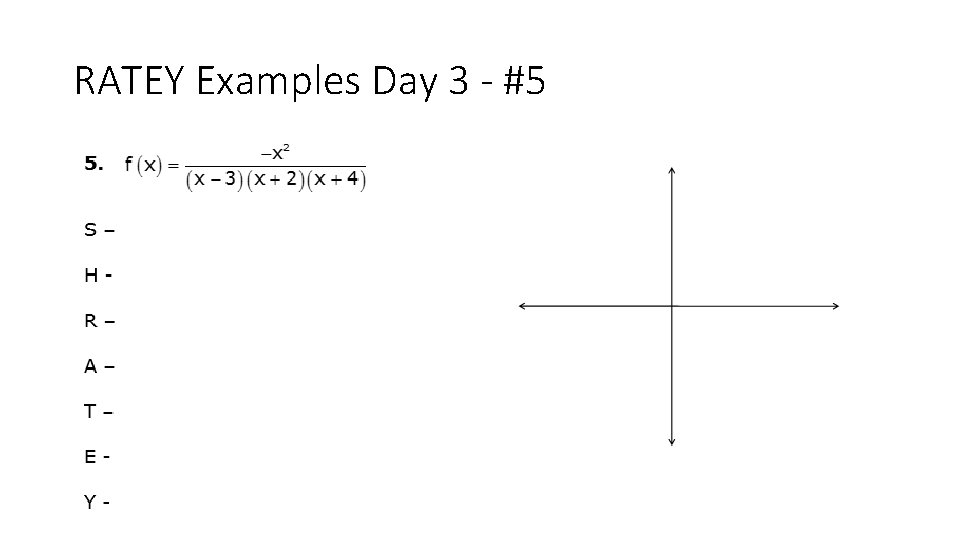RATEY Examples Day 3 - #5RATEY Examples Day 3 - #5 Already in simplest terms No factors cancel, so no holes -x 2=0 so x=0 Root at (0, 0) X-3=0 so x= 3 and x+2=0 so x= -2 and x+4=0 so x=-4 There are vertical asymptotes at x =3, x=-2 and x= -4 Graph is Tangent at (0, 0) “bottom heavy” The end behavior follows the line y = 0 Y=(-02)/((0 -3)(0+2)(0+4)) = 0 y-intercept is (0, 0)RATEY Examples Day 3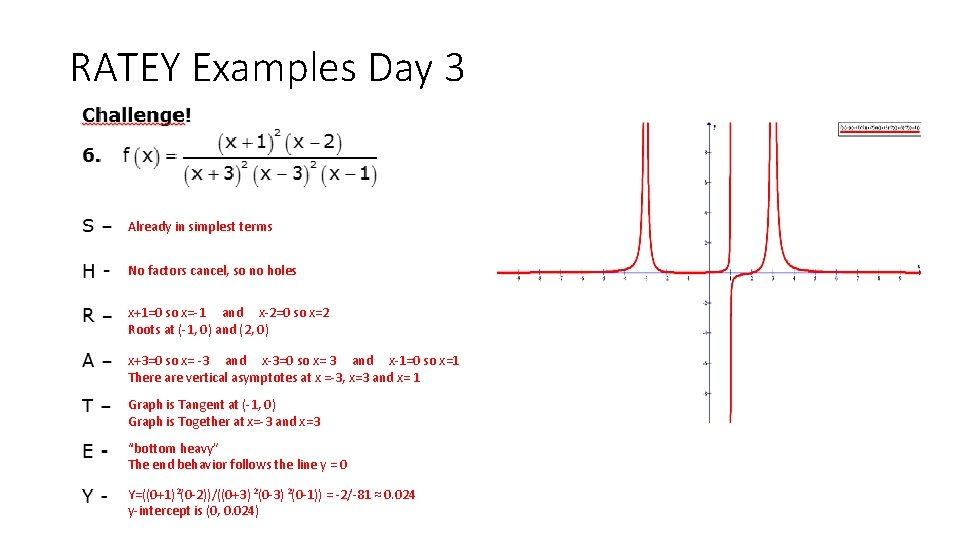RATEY Examples Day 3 Already in simplest terms No factors cancel, so no holes x+1=0 so x=-1 and x-2=0 so x=2 Roots at (-1, 0) and (2, 0) x+3=0 so x= -3 and x-3=0 so x= 3 and x-1=0 so x=1 There are vertical asymptotes at x =-3, x=3 and x= 1 Graph is Tangent at (-1, 0) Graph is Together at x=-3 and x=3 “bottom heavy” The end behavior follows the line y = 0 Y=((0+1)2(0 -2))/((0+3) 2(0 -1)) = -2/-81 ≈ 0. 024 y-intercept is (0, 0. 024)ASSIGNMENT Finish RATEY worksheets 1 -3 – Due TOMORROW